# 图像处理的阈值

## 数字图像处理 阈值分割

2011-12-07 16:12:10 honesty2008 阅读数 4636
• ###### matlab 基于阈值分割的区域生长的医学图像分割3讲

matlab 基于阈值分割的区域生长的医学图像分割3讲 </p>

3课时 23分钟 614人学习 刘昱显
免费试看① 选择一个T的初始估计值②用T分割图像，生成两组像素：G1由所有灰度值大

③ 对区域G1和G2中的所有像素计算平均灰度值µ1和µ2
④ 计算新的阈值⑤重复步骤②到④，直到逐次迭代所得的T值之差小于事先定义的参数T0

I=imread('images_chapter_10\Fig10.29(a).jpg');
subplot(2,2,1);imshow(I);title('原图');
subplot(2,2,2);imhist(I);title('原图的直方图');
% I=double(I);
%T 建议初始化为图像中最大强度值和最小亮度值的平均值
% T=0.5*(double(min(I(:)))+double(max(I(:))));
% T=mean(I(:));
T=0;%阈值初始化值
T0=0.5; %预定偏差大小
done=false;num=0;%迭代次数
while ~done
g=I>=T;
%由于g或者~g有可能为空,因此必须分情况处理
if(size(I(g),1)==0)T1=mean(I(~g));
elseif(size(I(~g),1)==0)T1=mean(I(g));
else T1=0.5*(mean(I(g))+mean(I(~g)));
end
done=abs(T-T1)<=T0;
T=T1;
num=num+1;
end
T=uint8(T);I2=I>=T;
%graythresh可以直接求出阈值,该函数采用Ostu方法,与通过迭代产生的阈值几乎没有区别.
%注意:由于graythresh产生的阈值已被归一化到范围[0,1]内,必须在使用时将其缩放到相应
%的范围,如uint8类图像,需要乘以255.
T1=graythresh(I)*255;
subplot(2,2,[3 4]);imshow(I2);title('基本全局阈值算法处理结果');
xlabel(['通过程序求得的阈值为',num2str(T),',迭代次数为',num2str(num)]);

## [Python图像处理] 七.图像阈值化处理及算法对比

2018-10-30 22:19:36 Eastmount 阅读数 8400
• ###### matlab 基于阈值分割的区域生长的医学图像分割3讲

matlab 基于阈值分割的区域生长的医学图像分割3讲 </p>

3课时 23分钟 614人学习 刘昱显
免费试看

PS：请求帮忙点个Star，哈哈，第一次使用Github，以后会分享更多代码，一起加油。

1.阈值化
2.二进制阈值化
3.反二进制阈值化
4.截断阈值化
5.反阈值化为0
6.阈值化为0

PS：文章参考自己以前系列图像处理文章及OpenCV库函数，同时部分参考网易云视频，推荐大家去学习。同时，本篇文章涉及到《计算机图形学》基础知识，请大家下来补充。

PSS：2019年1~2月作者参加了CSDN2018年博客评选，希望您能投出宝贵的一票。我是59号，Eastmount，杨秀璋。投票地址：https://bss.csdn.net/m/topic/blog_star2018/index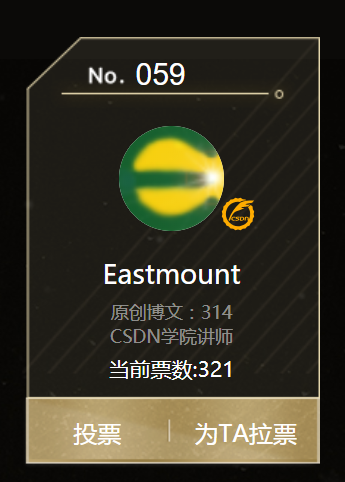# 一. 阈值化

（注：该部分参考作者的论文《基于苗族服饰的图像锐化和边缘提取技术研究》）

$\begin{cases} Y=0，gray=T\\ \end{cases}$

Python OpenCV中提供了阈值函数threshold()实现二值化处理，其公式及参数如下图所示：
retval, dst = cv2.threshold(src, thresh, maxval, type)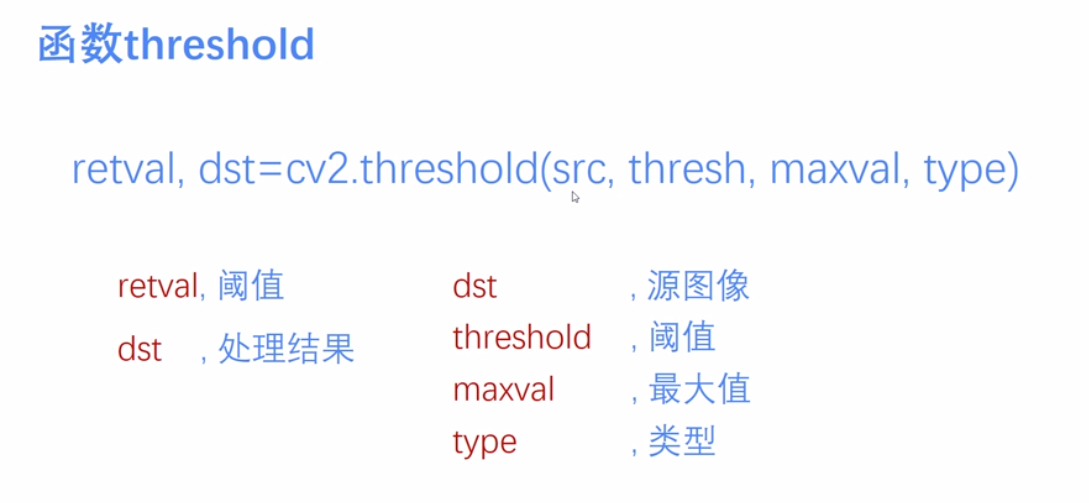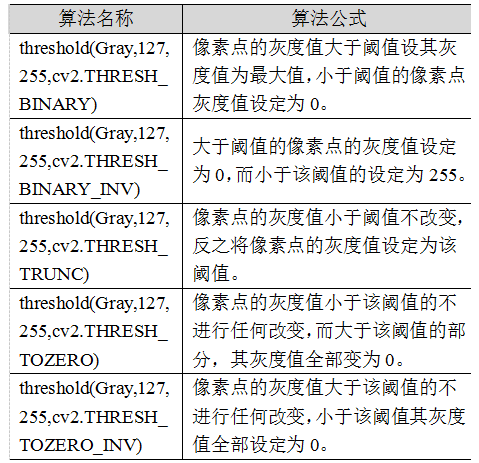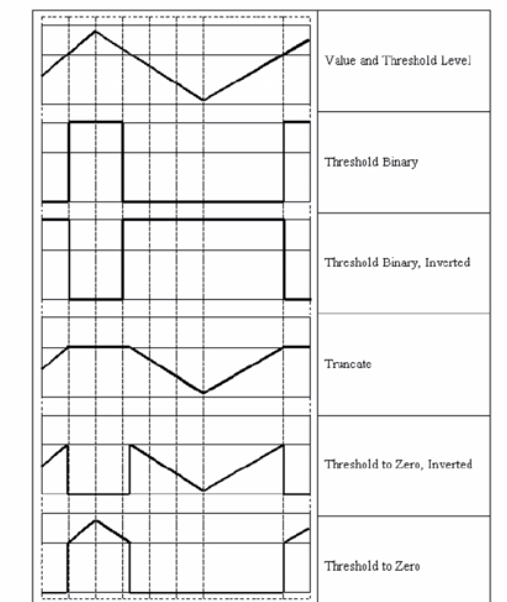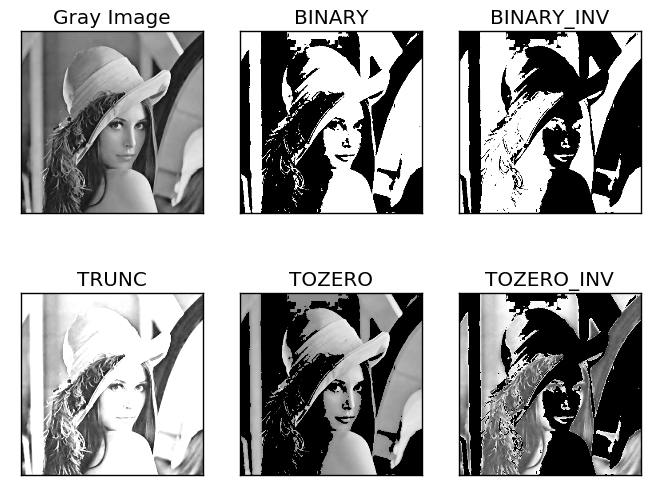# 二. 二进制阈值化

$dst(x,y) = \begin{cases} maxVal， if src(x,y)>thresh\\ 0，otherwise\\ \end{cases}$
(1) 大于等于127的像素点的灰度值设定为最大值（如8位灰度值最大为255）
(2) 灰度值小于127的像素点的灰度值设定为0

#encoding:utf-8
import cv2
import numpy as np

#读取图片

#灰度图像处理
GrayImage = cv2.cvtColor(src,cv2.COLOR_BGR2GRAY)

#二进制阈值化处理
r, b = cv2.threshold(GrayImage, 127, 255, cv2.THRESH_BINARY)
print r

#显示图像
cv2.imshow("src", src)
cv2.imshow("result", b)

#等待显示
cv2.waitKey(0)
cv2.destroyAllWindows()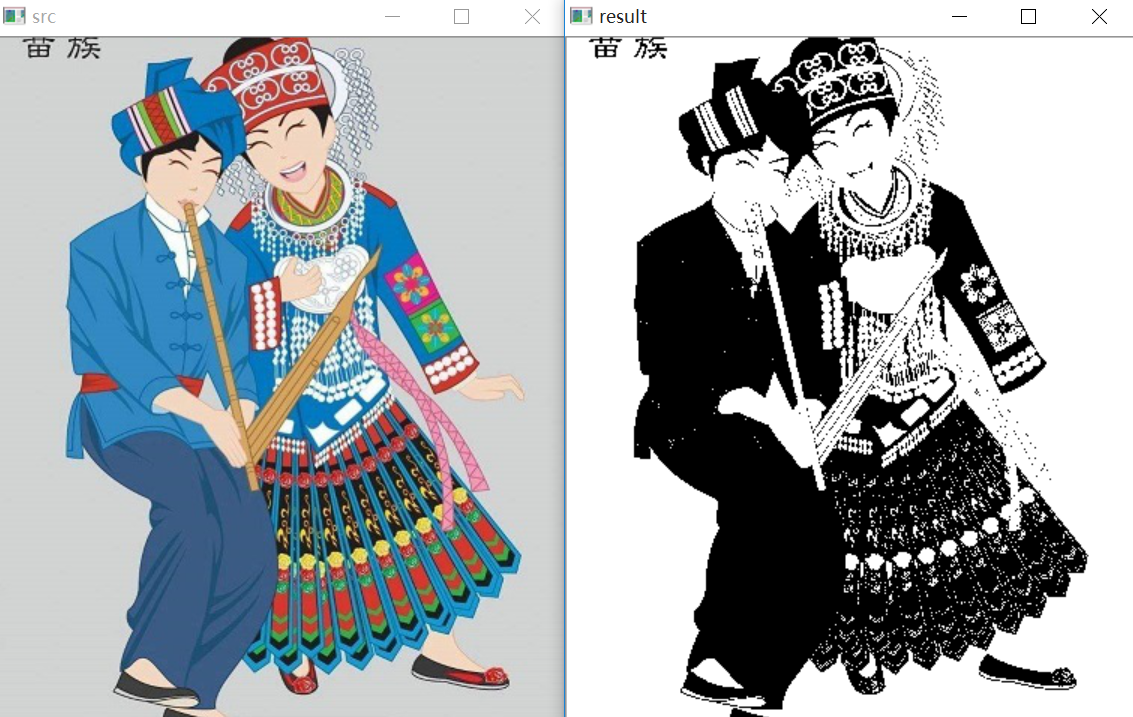# 三. 反二进制阈值化

$dst(x,y) = \begin{cases} 0， if src(x,y)>thresh\\ maxVal，otherwise\\ \end{cases}$
(1) 大于127的像素点的灰度值设定为0（以8位灰度图为例）
(2) 小于该阈值的灰度值设定为255

#encoding:utf-8
import cv2
import numpy as np

#读取图片

#灰度图像处理
GrayImage = cv2.cvtColor(src,cv2.COLOR_BGR2GRAY)

#反二进制阈值化处理
r, b = cv2.threshold(GrayImage, 127, 255, cv2.THRESH_BINARY_INV)
print r

#显示图像
cv2.imshow("src", src)
cv2.imshow("result", b)

#等待显示
cv2.waitKey(0)
cv2.destroyAllWindows()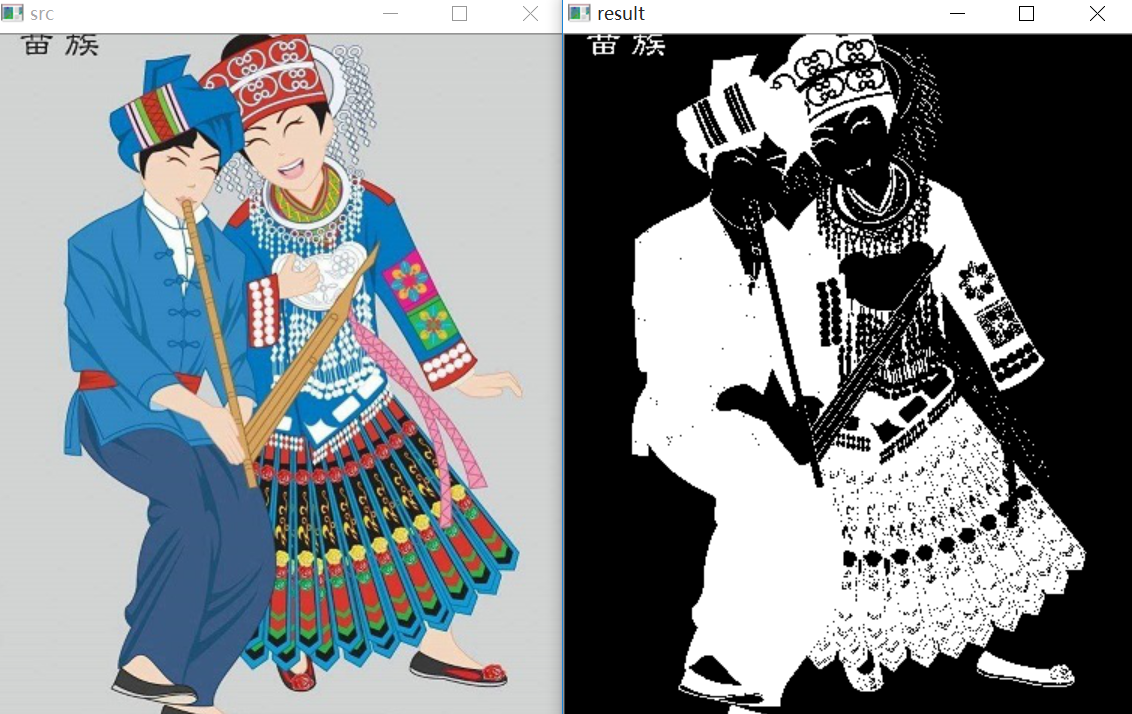# 四. 截断阈值化

$dst(x,y) = \begin{cases} threshold， if src(x,y)>thresh\\ src(x,y)，otherwise\\ \end{cases}$
(1) 大于等于127的像素点的灰度值设定为该阈值127
(2) 小于该阈值的灰度值不改变

#encoding:utf-8
import cv2
import numpy as np

#读取图片

#灰度图像处理
GrayImage = cv2.cvtColor(src,cv2.COLOR_BGR2GRAY)

#截断阈值化处理
r, b = cv2.threshold(GrayImage, 127, 255, cv2.THRESH_TRUNC)
print r

#显示图像
cv2.imshow("src", src)
cv2.imshow("result", b)

#等待显示
cv2.waitKey(0)
cv2.destroyAllWindows()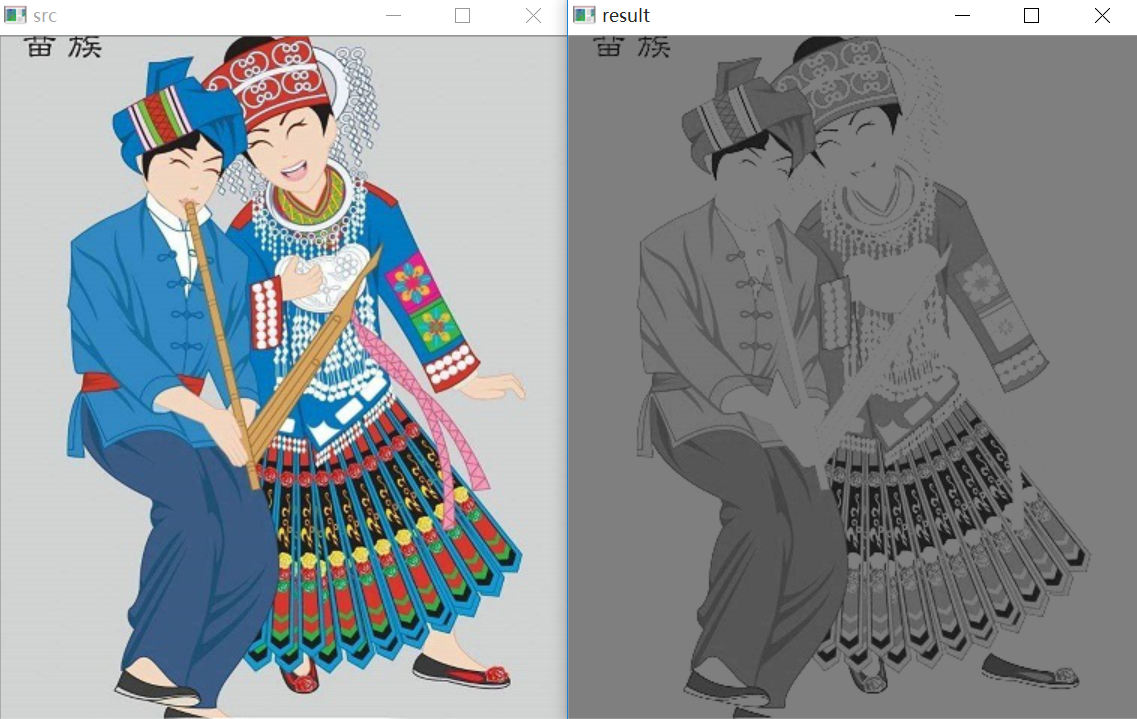# 五. 反阈值化为0

$dst(x,y) = \begin{cases} 0， if src(x,y)>thresh\\ src(x,y)，otherwise\\ \end{cases}$
(1) 大于等于阈值127的像素点变为0
(2) 小于该阈值的像素点值保持不变

#encoding:utf-8
import cv2
import numpy as np

#读取图片

#灰度图像处理
GrayImage = cv2.cvtColor(src,cv2.COLOR_BGR2GRAY)

#反阈值化为0处理
r, b = cv2.threshold(GrayImage, 127, 255, cv2.THRESH_TOZERO_INV)
print r

#显示图像
cv2.imshow("src", src)
cv2.imshow("result", b)

#等待显示
cv2.waitKey(0)
cv2.destroyAllWindows()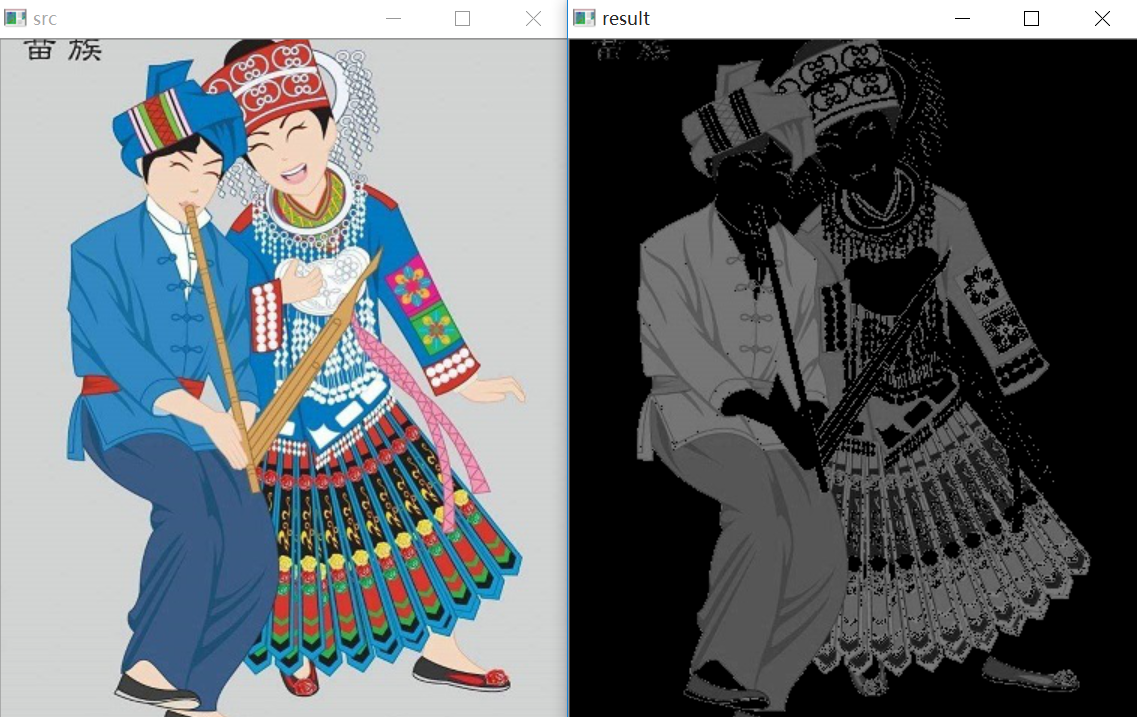# 六. 阈值化为0

$dst(x,y) = \begin{cases} src(x,y)， if src(x,y)>thresh\\ 0，otherwise\\ \end{cases}$
(1) 大于等于阈值127的像素点，值保持不变
(2) 小于该阈值的像素点值设置为0

#encoding:utf-8
import cv2
import numpy as np

#读取图片

#灰度图像处理
GrayImage = cv2.cvtColor(src,cv2.COLOR_BGR2GRAY)

#阈值化为0处理
r, b = cv2.threshold(GrayImage, 127, 255, cv2.THRESH_TOZERO)
print r

#显示图像
cv2.imshow("src", src)
cv2.imshow("result", b)

#等待显示
cv2.waitKey(0)
cv2.destroyAllWindows()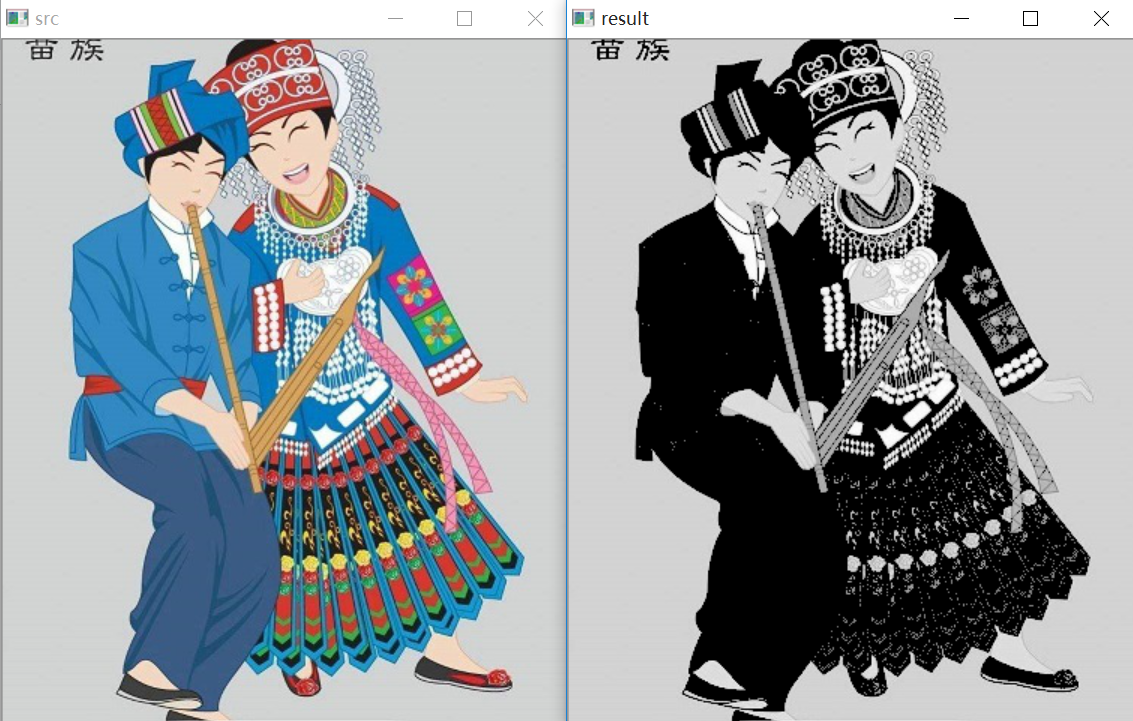#encoding:utf-8
import cv2
import numpy as np
import matplotlib.pyplot as plt

#读取图像
lenna_img = cv2.cvtColor(img,cv2.COLOR_BGR2RGB)
GrayImage=cv2.cvtColor(img,cv2.COLOR_BGR2GRAY)

#阈值化处理
ret,thresh1=cv2.threshold(GrayImage,127,255,cv2.THRESH_BINARY)
ret,thresh2=cv2.threshold(GrayImage,127,255,cv2.THRESH_BINARY_INV)
ret,thresh3=cv2.threshold(GrayImage,127,255,cv2.THRESH_TRUNC)
ret,thresh4=cv2.threshold(GrayImage,127,255,cv2.THRESH_TOZERO)
ret,thresh5=cv2.threshold(GrayImage,127,255,cv2.THRESH_TOZERO_INV)

#显示结果
titles = ['Gray Image','BINARY','BINARY_INV','TRUNC','TOZERO','TOZERO_INV']
images = [GrayImage, thresh1, thresh2, thresh3, thresh4, thresh5]
for i in xrange(6):
plt.subplot(2,3,i+1),plt.imshow(images[i],'gray')
plt.title(titles[i])
plt.xticks([]),plt.yticks([])
plt.show()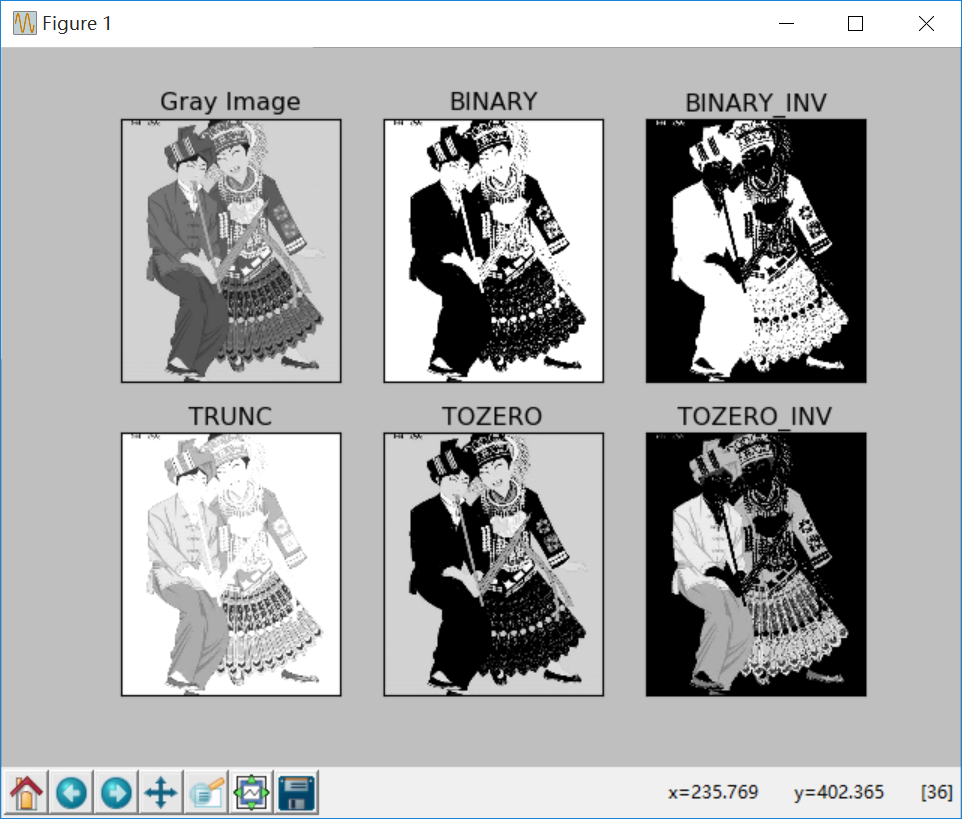（By：Eastmount 2018-10-30 晚上10点 https://blog.csdn.net/Eastmount/）

## 数字图像处理笔记——阈值（Thresholding）

2018-12-02 10:55:25 shanwenkang 阅读数 3276
• ###### matlab 基于阈值分割的区域生长的医学图像分割3讲

matlab 基于阈值分割的区域生长的医学图像分割3讲 </p>

3课时 23分钟 614人学习 刘昱显
免费试看

# 阈值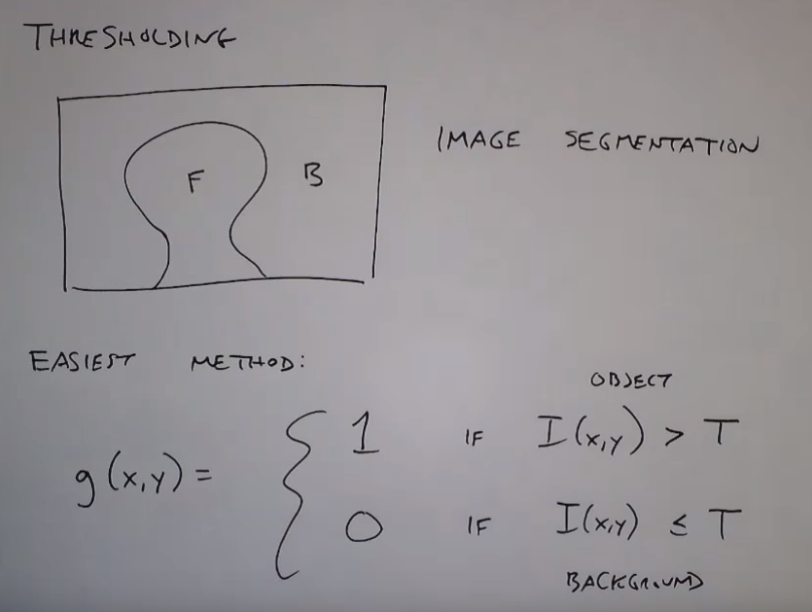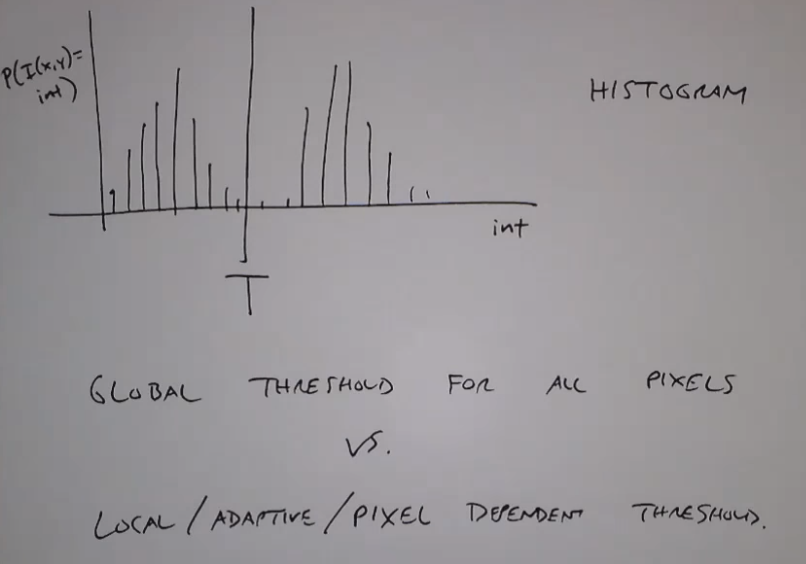# 全局阈值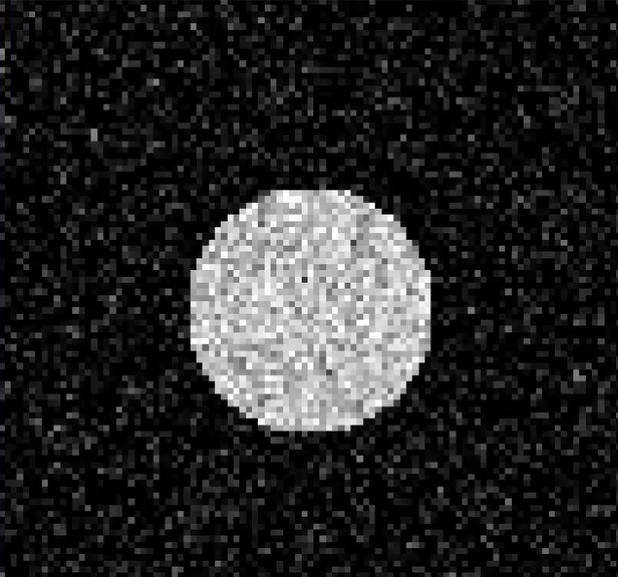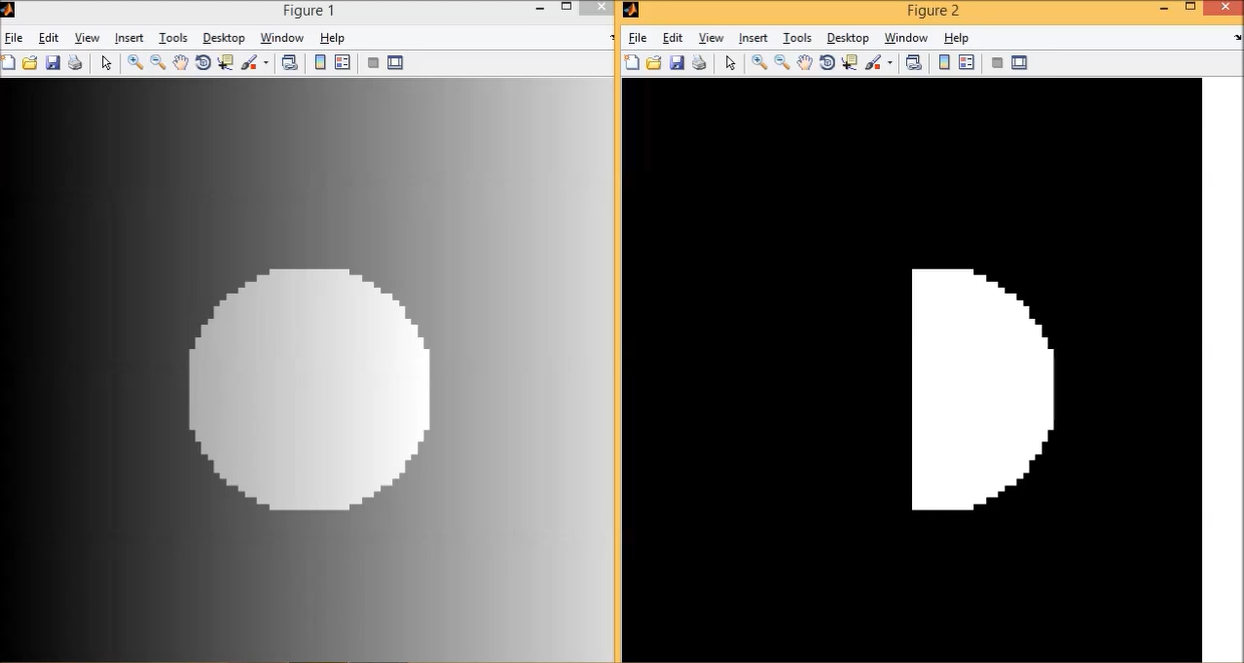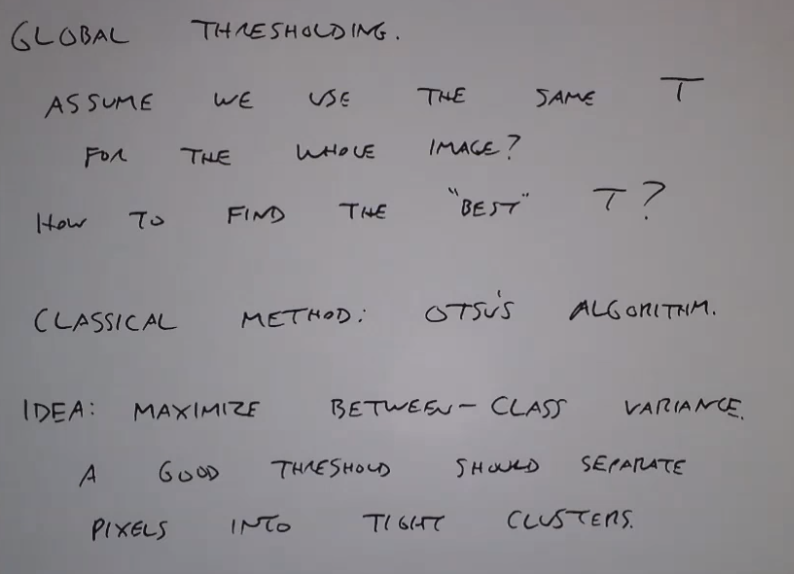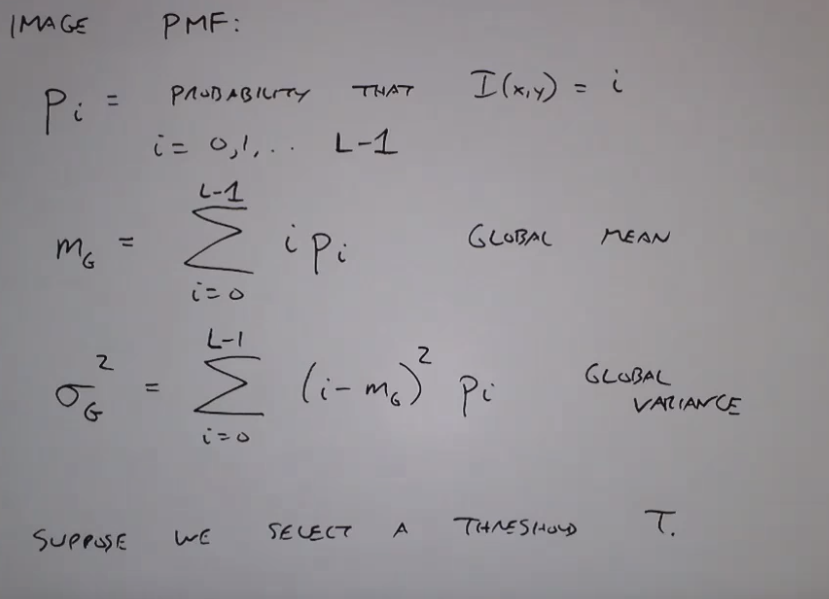OTSU算法就是想把图像分为两个块，然后使得这两个块之间的方差最大，也就是最大化两个块的均值与全局均值的差的平方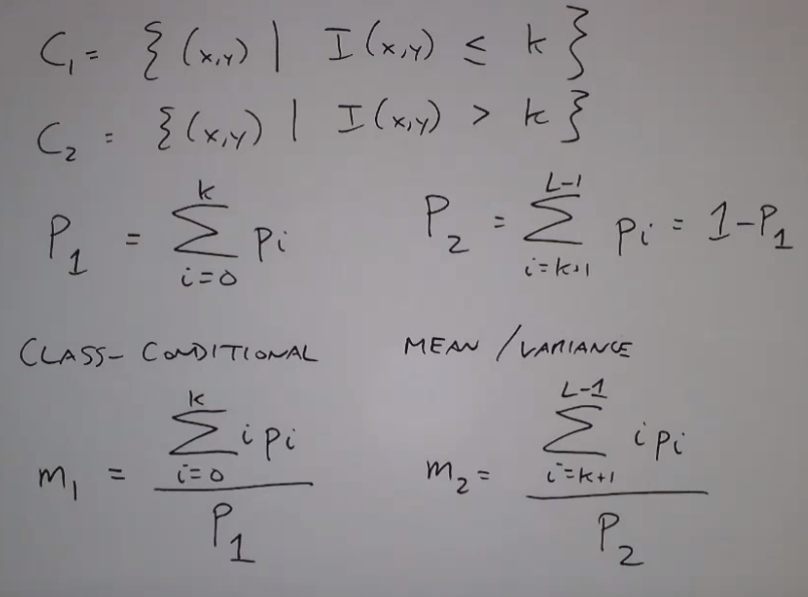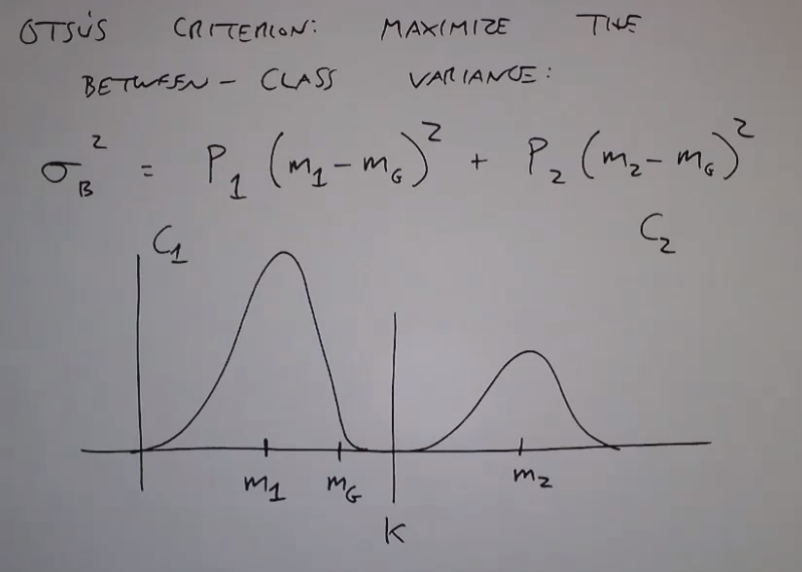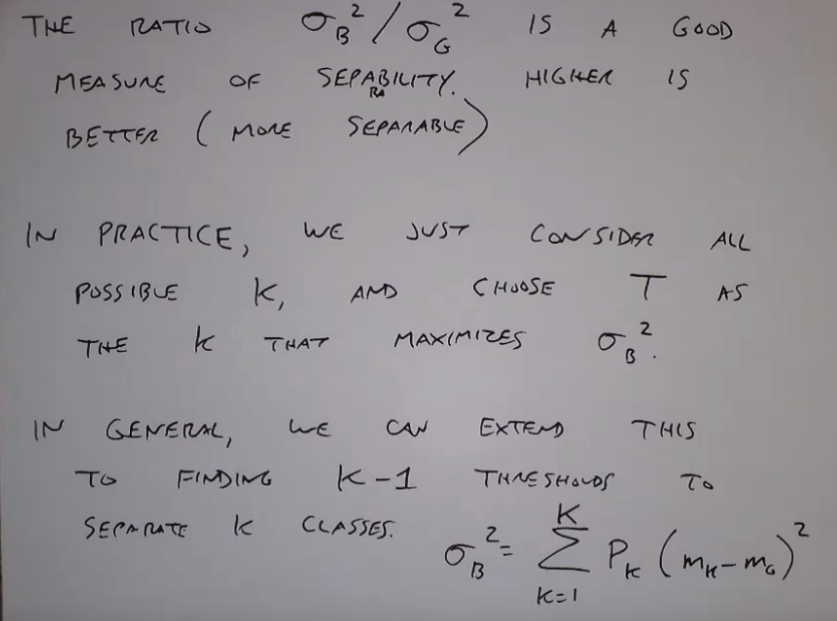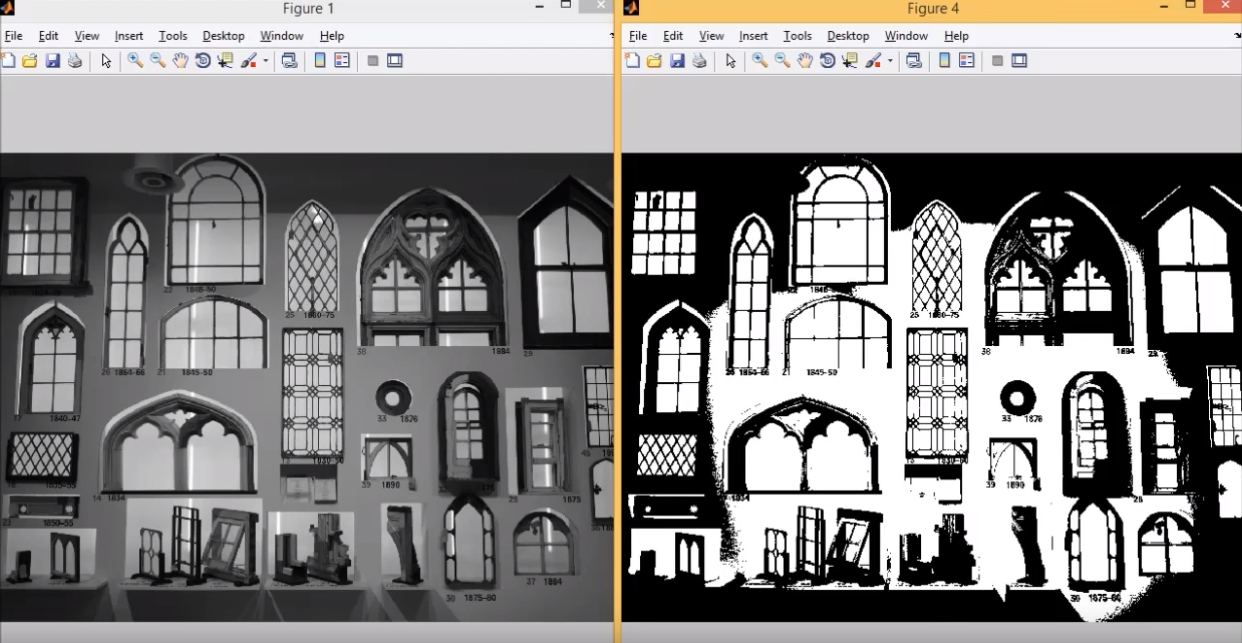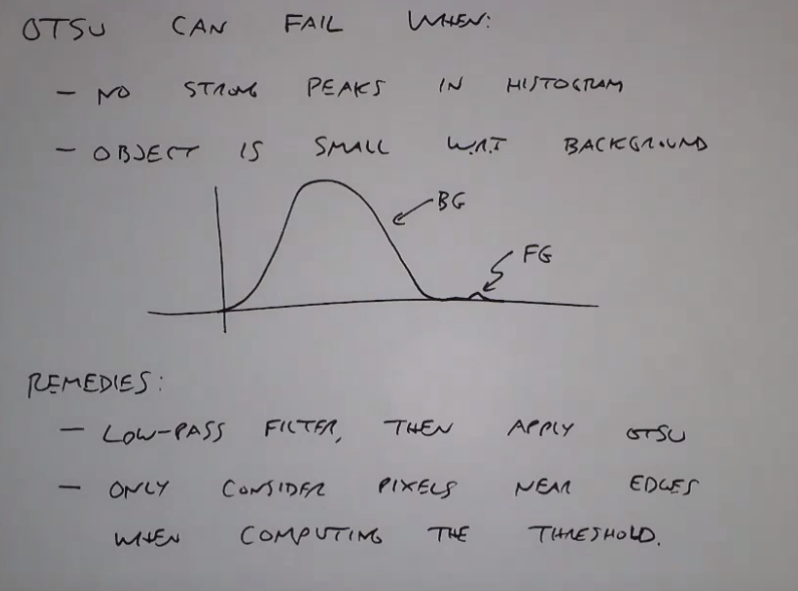## 局部阈值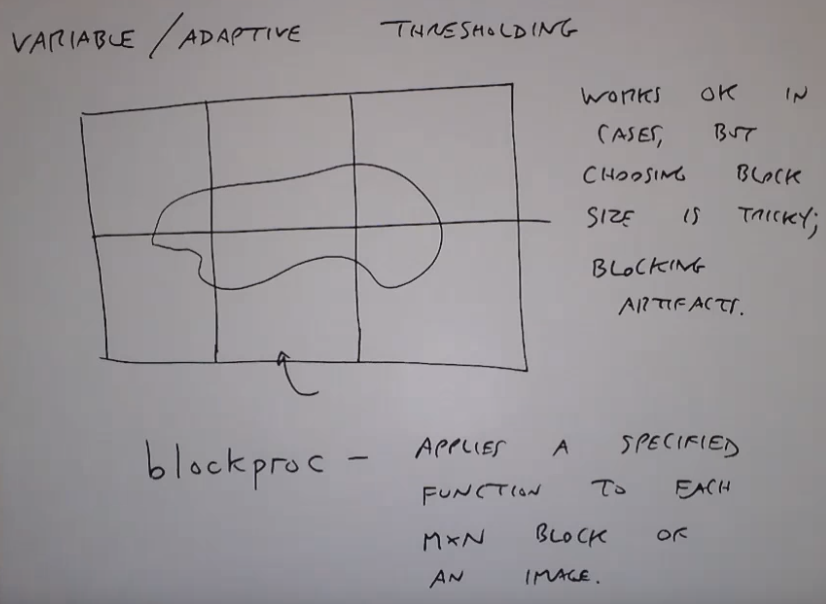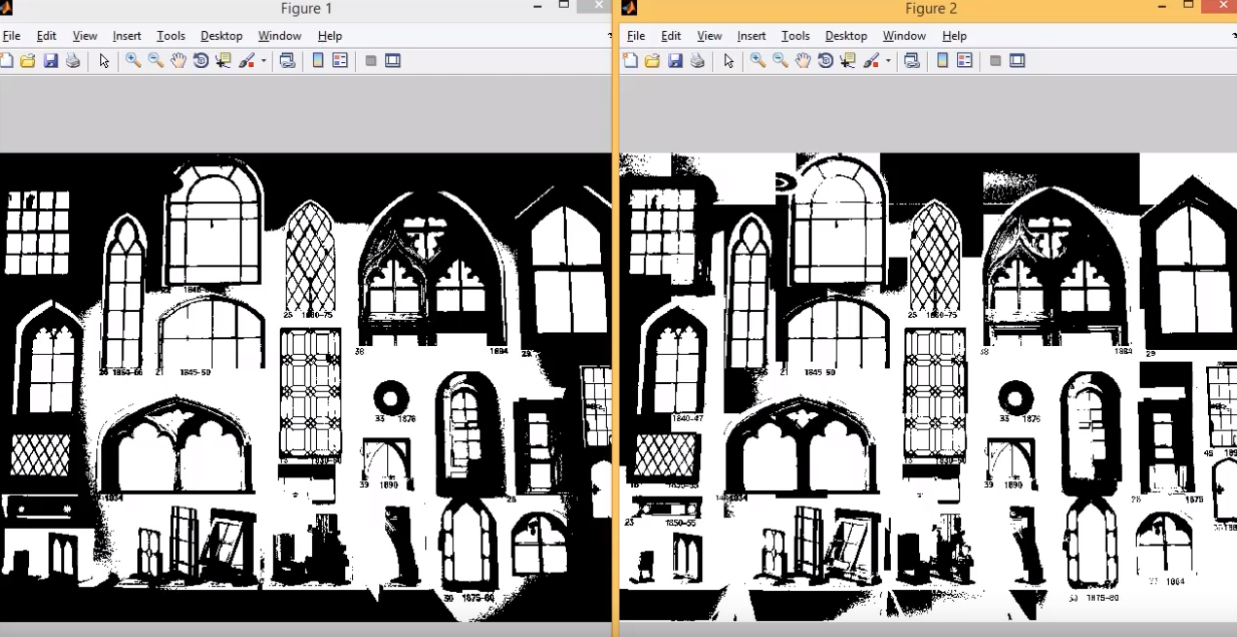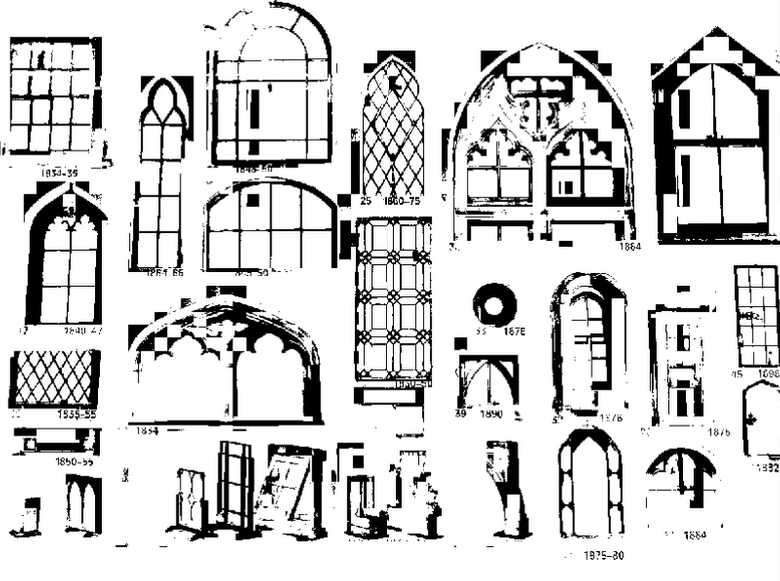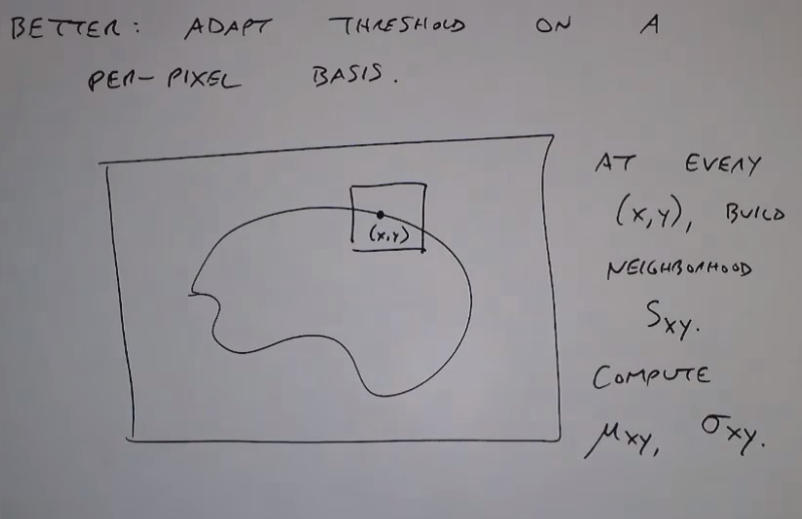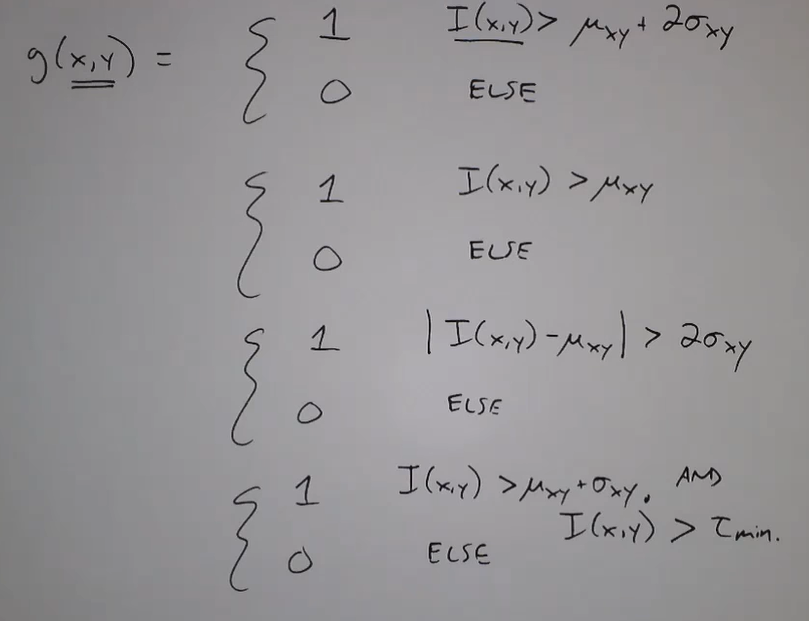### RGB图阈值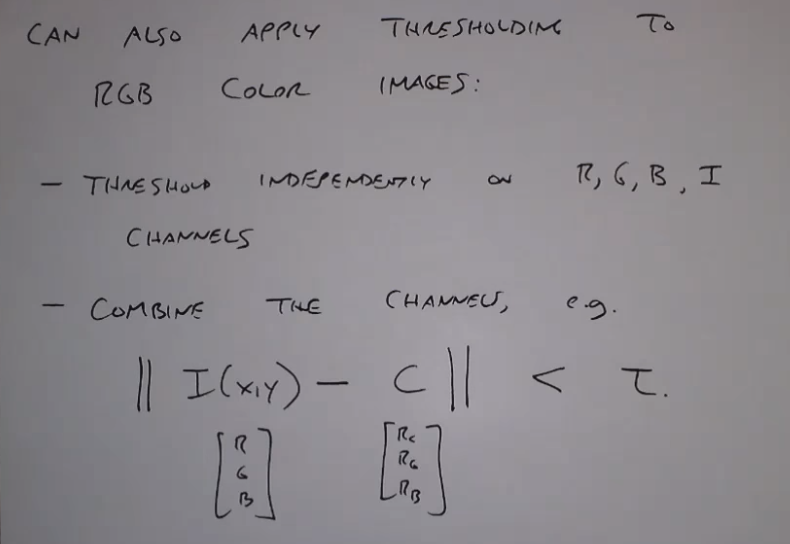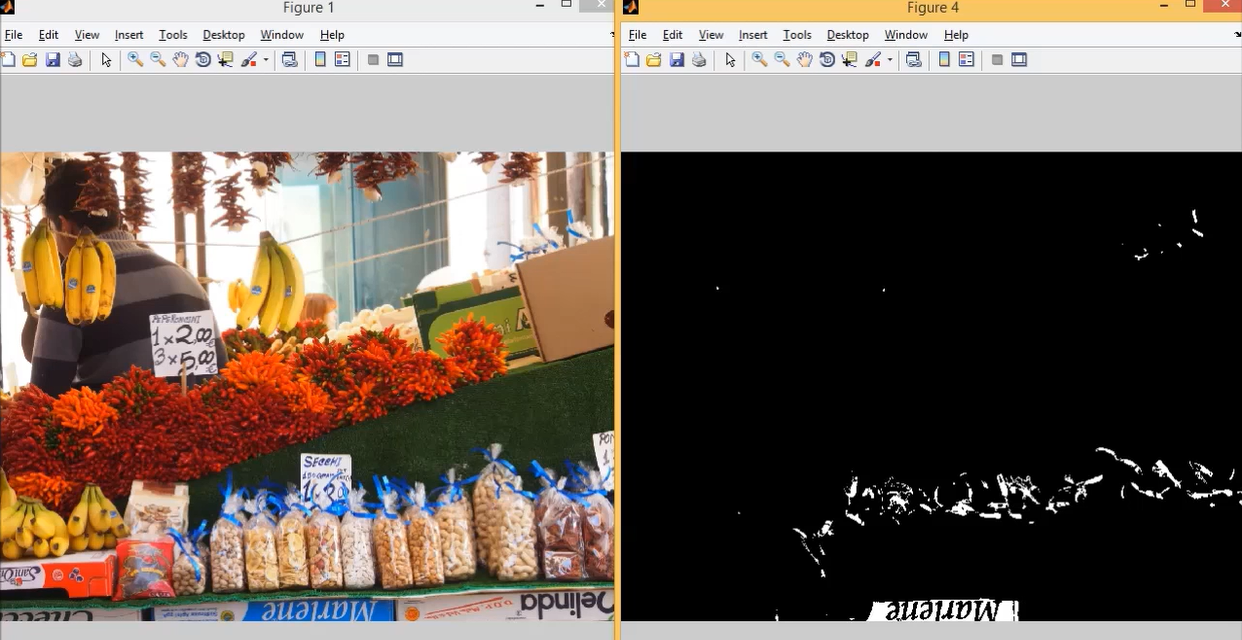## 在图像处理中阈值是什么意思？

2018-03-29 14:18:43 bjbz_cxy 阅读数 17248
• ###### matlab 基于阈值分割的区域生长的医学图像分割3讲

matlab 基于阈值分割的区域生长的医学图像分割3讲 </p>

3课时 23分钟 614人学习 刘昱显
免费试看
的意思是界限，故阈值又叫临界值，是指一个效应能够产生的最低值或最高值。
在图像处理中它的意思是颜色转换的临界点，该方法只用于二值化的图像中
列如在自然中每一种颜色都有一个值，通常由RGB（即红、绿、蓝三原色）按比例混合就会得到各种不同的颜色。阈值处理图片是对颜色进行特殊处理的一种方法。
详细说，阈值是一个转换临界点，不管你的图片是什么样的彩色，它最终都会把图片当黑白图片处理，也就是说你设定了一个阈值之后，它会以此值作标准，凡是比该值大的颜色就会转换成白色，低于该值的颜色就转换成黑色，所以最后的结果是，你得到一张黑白的图片。
用阈值的作用：得到一张对比度不同的黑白图片

## 图像处理（阈值篇）

2019-10-17 19:34:58 weixin_43384504 阅读数 306
• ###### matlab 基于阈值分割的区域生长的医学图像分割3讲

matlab 基于阈值分割的区域生长的医学图像分割3讲 </p>

3课时 23分钟 614人学习 刘昱显
免费试看

1.直方图分割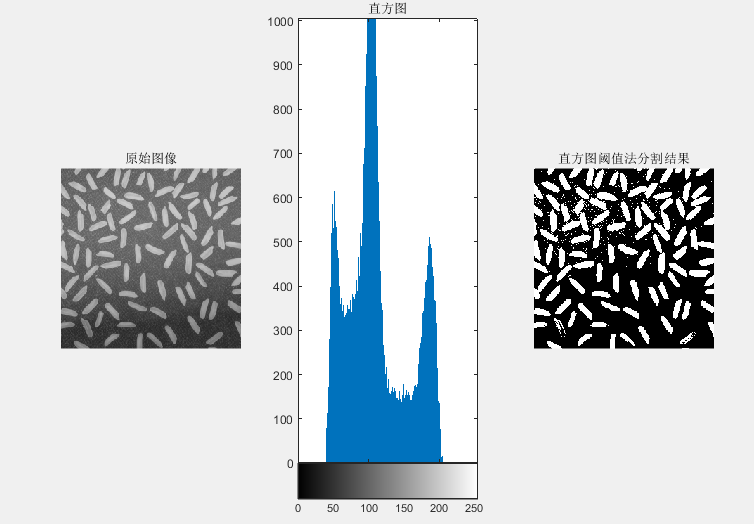clear all
close all
figure, subplot(1,3,1);
imshow(I),title('原始图像')
subplot(1,3,2);
imhist(I),title('直方图')   % 观察灰度直方图
I1=im2bw(I,120/255); % im2bw 函数需要将灰度值转换到[0,1]范围内
subplot(1,3,3);

120就是谷底的位置。

2.全局分割（OTSU）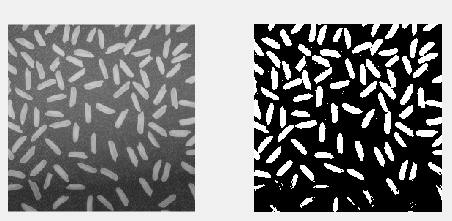% I=imread('rice.png');
% figure,subplot(1,2,1);
% imshow(I);
% s=imbinarize(I);
% subplot(1,2,2);
% imshow(s);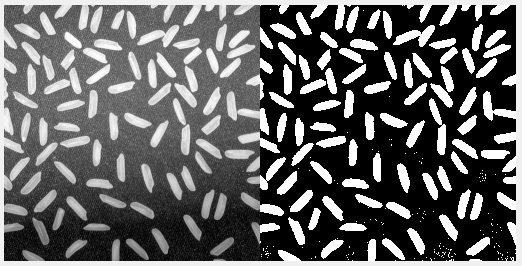I = imread('rice.png');
figure
imshowpair(I,BW,'montage')

1.分水岭的方法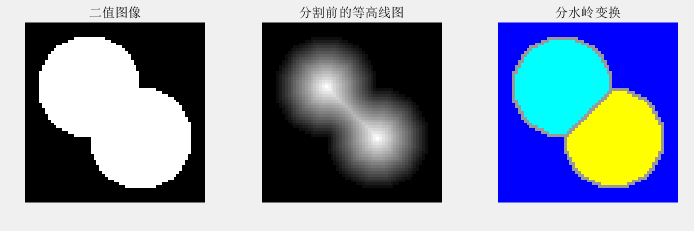clear all;
ct1 = -9;
ct2 = -ct1;
dist = sqrt(2*(2*ct1)^2);
ra = dist/2 * 1.4;
lims = [floor(ct1-1.2*ra) ceil(ct2+1.2*ra)];
[x,y] = meshgrid(lims(1):lims(2));
bw1 = sqrt((x-ct1).^2 + (y-ct1).^2) <= ra;
bw2 = sqrt((x-ct2).^2 + (y-ct2).^2) <= ra;
bw = bw1 | bw2;
subplot(131), imshow(bw,'InitialMagnification','fit'),
title('二值图像');
F = bwdist(~bw);
subplot(132), imshow(F,[],'InitialMagnification','fit')
title('分割前的等高线图');
F = -F;
F(~bw) = -Inf;
%进行watershed分割并将分割结果以标记图形式绘出
L = watershed(F);
rgb = label2rgb(L,'jet',[.6 .6 .6]);
subplot(133), imshow(rgb,'InitialMagnification','fit')
title('分水岭变换')


matlab 中以默认以欧式距离作为判断的标准。这个可以自己去改。

2.k-means聚类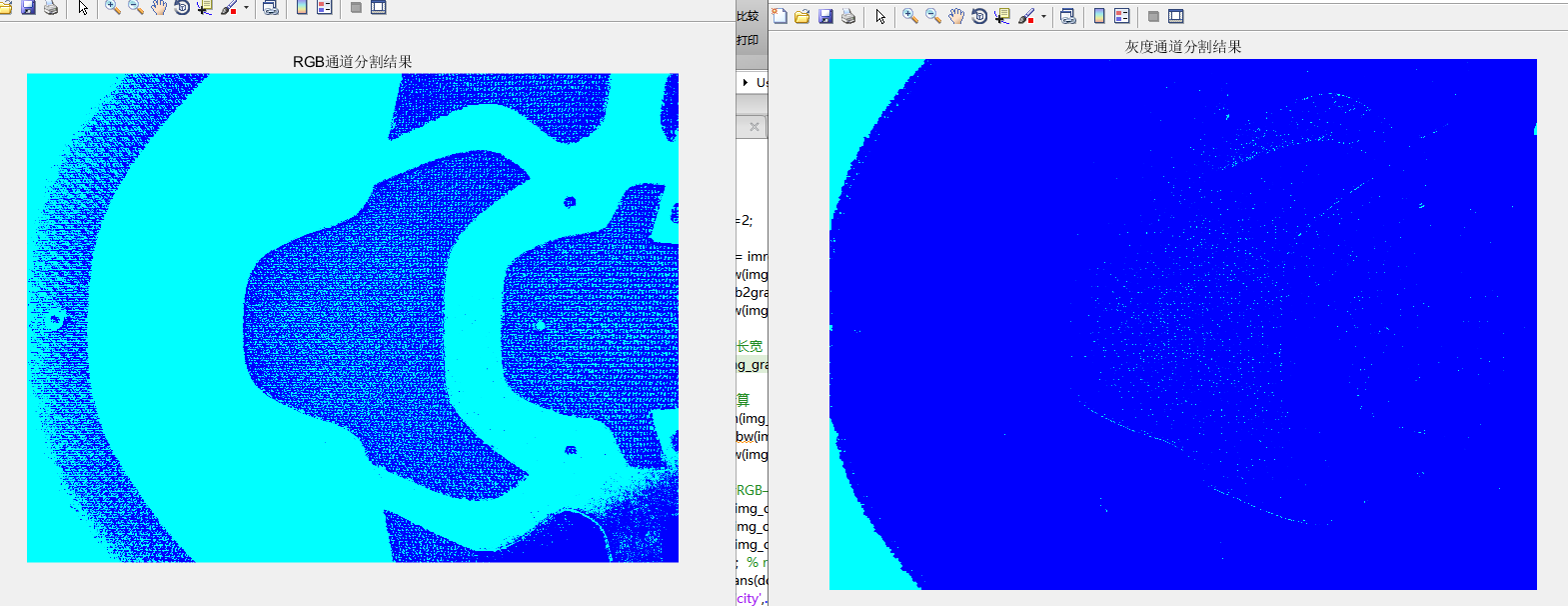简单的看一下结果，彩色通道效果还不错的样子。

clc;

C_Segments=2;

figure,imshow(img_original),title('原始图像');    %显示原图像
img_gray=rgb2gray(img_original);
figure,imshow(img_gray),title('原始灰度图像');

% 获取图像的长宽
[m,n]=size(img_gray);

% 灰度阈值计算
T=graythresh(img_gray);
img_bw=im2bw(img_gray,T);
figure,imshow(img_bw),title('原始二值图像');

% 将图像进行RGB——3通道分解
A = reshape(img_original(:, :, 1), m*n, 1);    % 将RGB分量各转为kmeans使用的数据格式n行，一样一样本
B = reshape(img_original(:, :, 2), m*n, 1);
C = reshape(img_original(:, :, 3), m*n, 1);
dat = [A B C];  % r g b分量组成样本的特征，每个样本有三个属性值，共width*height个样本
cRGB = kmeans(double(dat), C_Segments,...
'Distance','city',...
'emptyaction','singleton',...
'start','sample');    % 使用聚类算法分为2类
rRGB = reshape(cRGB, m, n);     % 反向转化为图片形式
figure, imshow(label2rgb(rRGB)),title('RGB通道分割结果');   % 显示分割结果

% 将图像进行单一通道灰度分解
GraySeg= reshape(img_gray(:, :), m*n, 1);
cGray=kmeans(double(GraySeg), 2);
rGray= reshape(cGray, m, n);     % 反向转化为图片形式
figure, imshow(label2rgb(rGray)),title('灰度通道分割结果');   % 显示分割结果